Function Repository Resource:

# TimeSeriesCompress

Remove redundant data from a time series

Contributed by: Sascha Kratky
 ResourceFunction["TimeSeriesCompress"][tseries] compresses time series tseries by removing data points that can be accurately predicted through linear interpolation.

## Details and Options

ResourceFunction["TimeSeriesCompress"] is used to remove redundant data points from a time series. The resulting time series requires less memory, but behaves as the original series within given accuracy requirements.
The input tseries can be a list of values {x1,x2,}, a list of time-value pairs {{t1,x1},{t2,x2},}, a TimeSeries, an EventSeries or TemporalData.
If times are not given, then tseries is assumed to be regular with unit spacing.
ResourceFunction["TimeSeriesCompress"] uses a default tolerance for time series values of 10-10.
ResourceFunction["TimeSeriesCompress"] uses a maximal allowed time distance of ∞.
ResourceFunction["TimeSeriesCompress"] removes Missing data points as a side effect of compression.
ResourceFunction["TimeSeriesCompress"] takes the following options:
 "ValueTolerance" 10-10 maximal allowed value deviation with respect to the original value "MaxTimeDistance" Infinity maximal allowed time distance between two data points

## Examples

### Basic Examples (4)

Create an example time series:

 In:=Out=Visualize the path:

 In:=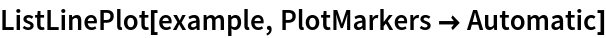Out=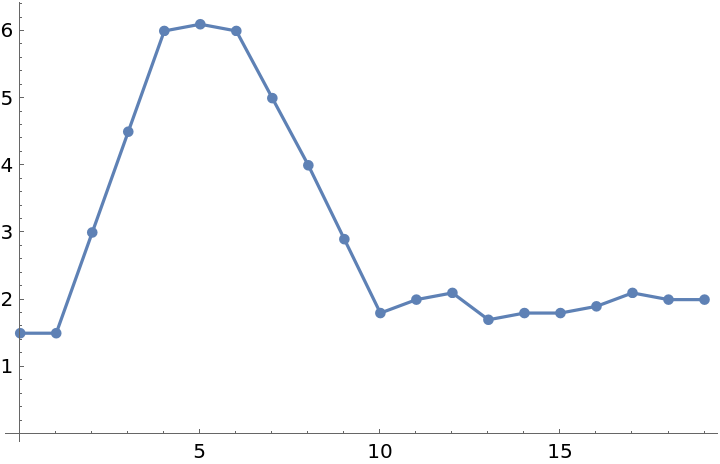Four redundant points are removed from the time series by compression:

 In:=Out=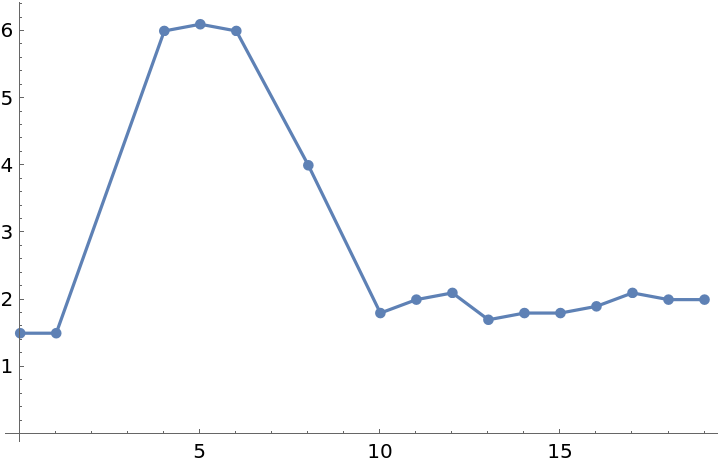Visualize the removed points:

 In:=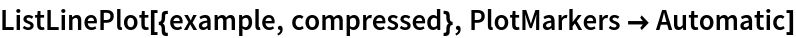Out=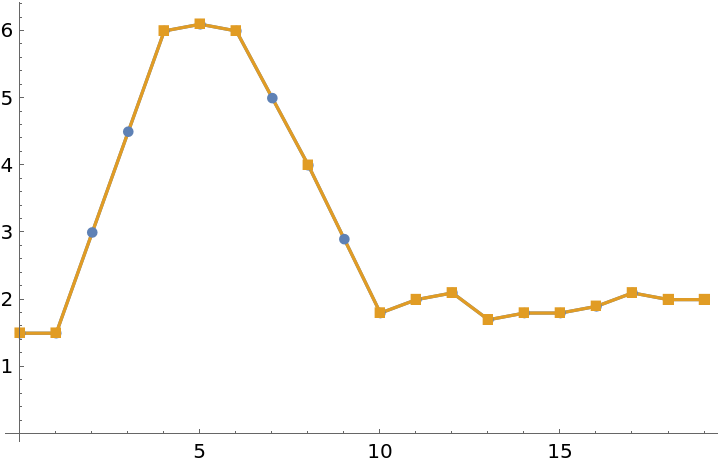### Scope (3)

Compress a list of numeric values:

 In:=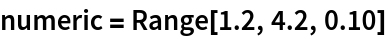Out=In:=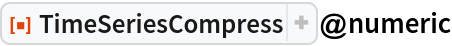Out=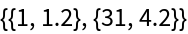Compress a list of time-value pairs:

 In:=In:=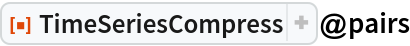Out=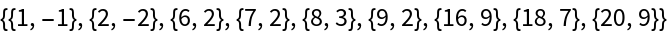Compressing TemporalData applies compression to all underlying paths. The resampling is changed to linear interpolation:

 In:=Out=### Options (4)

Increasing the maximal allowed deviation in the time series value leads to better compression:

 In:=Out=In:=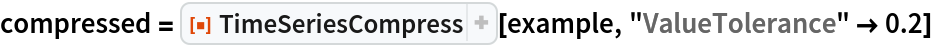Out=Visualize the removed points:

 In:=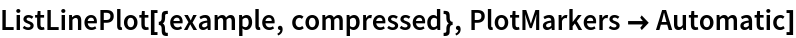Out=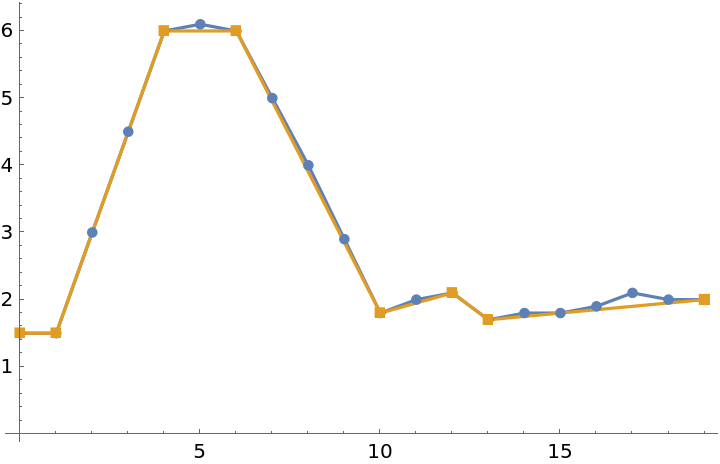Backtesting the compressed time series values with the original time series values:

 In:=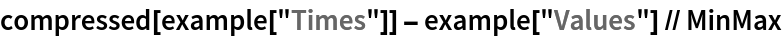Out=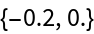Limit the maximal time distance between two points:

 In:=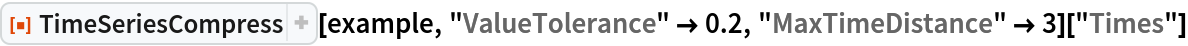Out=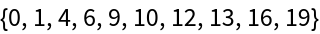### Possible Issues (3)

TimeSeriesCompress only works on time series whose values are scalars. Time series with higher-dimensional values are returned uncompressed:

 In:=Out=EventSeries is a special case of TemporalData allowing no interpolation. To compress an EventSeries, convert it to a TimeSeries:

 In:=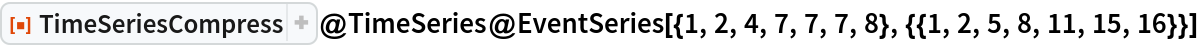Out=TimeSeriesCompress removes values with head Missing:

 In:=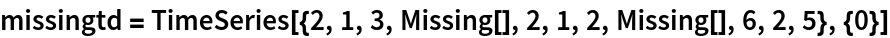Out=In:=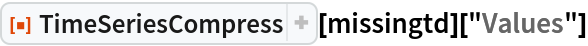Out=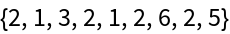### Neat Examples (3)

Use TemporalData to store the stock prices of the FAANG companies since the beginning of the decade:

 In:=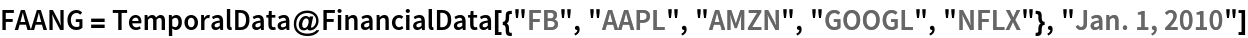Out=If you are not interested in cent fluctuations of the prices of these stocks, you can work with a compressed representation:

 In:=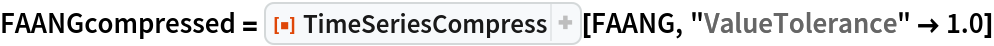Out=Compression reduces the required data points by about 40 percent:

 In:=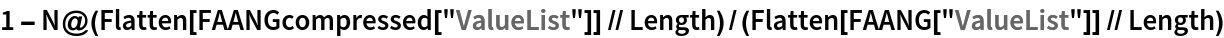Out=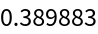Sascha Kratky

## Version History

• 1.0.0 – 07 October 2019